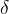# Two-Body Problem by the Singular Rank One Nonsymmetric Perturbation

## Authors

• Тетяна Іванівна Вдовенко NTUU KPI, Ukraine

## Keywords:

Singular perturbations, Nonsymmetrical perturbations, Nonlocal interaction, The problem of two bodies

## Abstract

Background. We consider nonself-adjoint singular perturbation of rank one of a self-adjoint operator by nonsymmetrical potential, i.e., the formal expression $\tilde{A}=A+\alpha \left \langle \cdot ,\omega _{1} \right \rangle\omega _{2},$ where A is semibounded self-adjoint operator in the separable Hilbert space  $\mathfrak{H}, \alpha \in \mathbb{C}.$ In compare with many previous studies of self-adjoint perturbation, the vectors $\omega _{1}, \omega _{2}\in \mathfrak{H_{-2}}$ are different, i.e., $\omega _{1} \neq \omega _{2},$ that is some general problem about nonlocal interactions. The additional difficulties are that the Hilbert space H has a form of tensor product of spaces $H=\mathfrak{K} \otimes \mathfrak{H},$ and the operator has a form $\mathfrak{A}=\mathit{B}\otimes I_{\mathfrak{H}}+I_{\mathfrak{K}}\otimes \mathbf{\mathit{A}},$ which together illustrate the problem of two bodies.

Objective. The purpose of research in our work is the description of singular perturbation of operator of the form $\mathfrak{\tilde{A}}=B\otimes I_{\mathfrak{N}}+I_{\mathfrak{K}}\otimes \tilde{A},$ where $\tilde{A}$ is a self-adjoint operator that is singularly perturbed by nonsymmetric potential of rank one.

Methods. We use the assertion of about the presentation in the resolvent form of a rank one singular perturbation of a self-adjoint operator by symmetric potential, which corresponds to the problem of two bodies, and the definition of the operator singularly rank one perturbed by nonsymmetric potential $\tilde{A}.$

Results. The representation of the operator $\mathfrak{\tilde{A}},$ given by formal expression in the form of resolvent is our main result.

Conclusions. We present the resolvent form for singularly perturbed self-adjoint rank one operator, which perturbed by nonsymmetric potential, appropriate the problem of two bodies. By representation we take into account the case when the perturbed operator requires additional parameterization. We look for the application of the general results to describe the problem with the use of two bodiesLaplace operator perturbed by nonsymmetric potentials composed of-functions Dirac in  $\mathbb{R}^{n}, n=2, 3.$

## Author Biography

### Тетяна Іванівна Вдовенко, NTUU KPI

Tetiana I. Vdovenko,

PhD student

## References

S. Albeverio and P. Kurasov, “Singular perturbations of differential operators. Solvable Schrödinger type operators”, in Lon­don Math. Soc. Lecture Note, Ser. 271. Cambridge, GB: Cambridge University Press, 2000.

S. Albeverio et al., Solvable Models in Quantum Mechanics, 2nd ed., appendix by P. Exner. Providence, RI: AMS Chelsea Publishing, 2005, 488 p.

Y. Berezansky and J. Brasche, “Generalized selfadjoint operators and their singular perturbations”, Methods Funct. Anal. Topology, vol. 7, no. 3, pp. 54–66, 2001.

V. Koshmanenko, “Singular quadratic forms in perturbation theory”, Mathematics and its Applications, vol. 474, pp. viii + 308, 1999.

Т.І. Vdovenko and М.E. Dudkin, “Singular nonsymmetric rank one perturbation of selfadjoint operator. Spectral theory of operators and sets of operators”, Zbirnyk Prats Instytutu Matematyky NAN Ukrainy, vol. 12, no. 1, pp. 57–73, 2015 (in Ukrainian).

Т.І. Vdovenko and М.E. Dudkin, “Wave operators for singular nonsymmetric perturbation of selfadjoint operator”, Bukovyns'kyy Matematychnyy Zhurnal, vol. 3 , no. 1, pp. 25–29, 2015 (in Ukrainian).

L. Nizhnik, “Inverse nonlocal Sturm-Liouville problem”, Inverse Problems, vol. 26, pp. 523–535, 2010.

L. Nizhnik, “Inverse spectral nonlocal problem for the first order ordinary differential equation”, Tamkang J. Math., vol. 42, no. 3, pp. 385–394, 2011.

M.E. Dudkin and V.D. Koshmanenko, Methods of Equipped Space in the Theory of Singular Perturbations of Selfadjoint Operator.Kyiv,Ukraine:InstituteofMathematicsof NASU, 2013, vol. 96 (Ser. Proc. Inst. Math. NASU) (in Ukrainian).

Т.І. Vdovenko, “Wave operators of a rank one singular perturbation by nonsymmetric potential”, Naukovi Visti NTUU KPI, no. 4, pp. 13–17, 2015 (in Ukrainian).